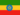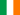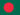# Alisamento exemplo-1

• Nota de Estudos
• Rever TópicosHenock A. 0 0 doneRachel N. 0 0 Smoothing - example 1Jahidul I. 0 0 Using the naïve method, all forecasts for the future are equal to the last observed value of the series, y^T+h|T=yT, for h=1,2,…. Hence, the naïve method assumes that the most current observation is the only important one and all previous observations provide no information for the future. This can be thought of as a weighted average where all the weight is given to the last observation. Using the average method, all future forecasts are equal to a simple average of the observed data, y^T+h|T=1T∑t=1Tyt, for h=1,2,…. Hence, the average method assumes that all observations are of equal importance and they are given equal weight when generating forecasts. We often want something between these two extremes. For example it may be sensible to attach larger weights to more recent observations than to observations from the distant past. This is exactly the concept behind simple exponential smoothing. Forecasts are calculated using weighted averages where the weights decrease exponentially as observations come from further in the past --- the smallest weights are associated with the oldest observations: y^T+1|T=αyT+α(1−α)yT−1+α(1−α)2yT−2+⋯,(7.1) where 0≤α≤1 is the smoothing parameter. The one-step-ahead forecast for time T+1 is a weighted average of all the observations in the series y1,…,yT. The rate at which the weights decrease is controlled by the parameter α.Jahidul I. 0 0 what is seasonal adjustment ?
• Text Version### Você recebeu uma nova notificação

Clique aqui para visualizar todos eles